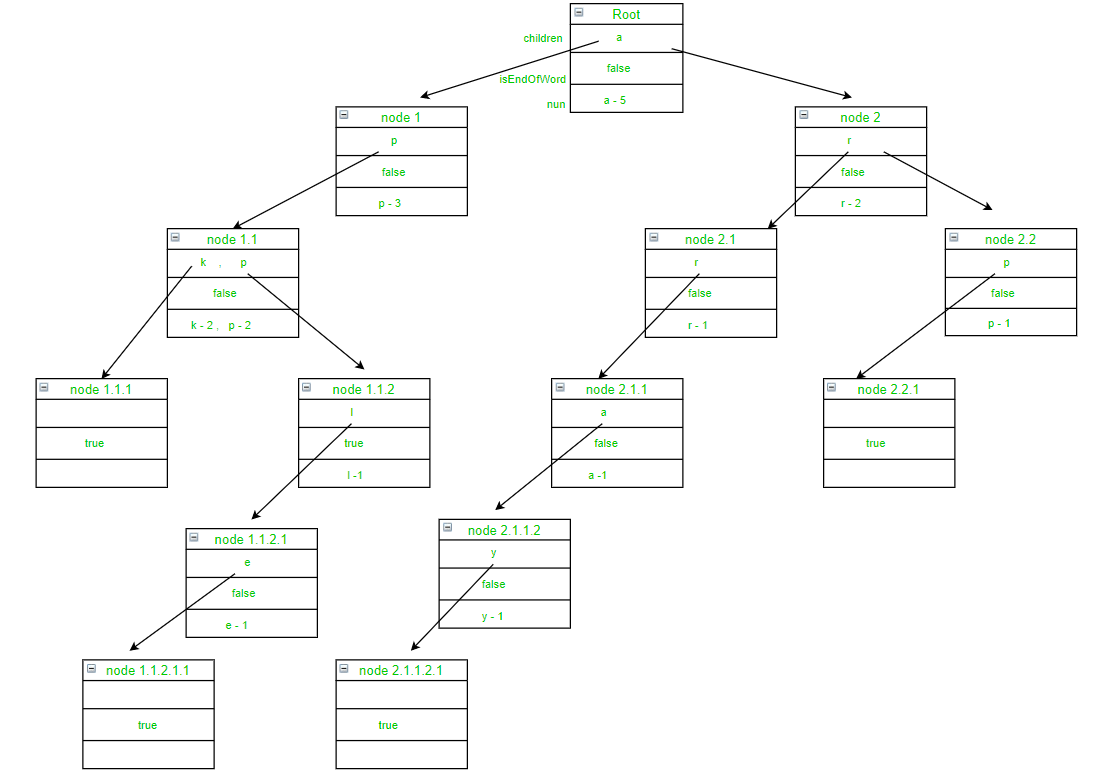# Count the number of words with given prefix using Trie

Prerequisite: Trie
Given a list of string str[] and a prefix string pre. The task is to count the number of words in list of string with given prefix using trie.

Examples:

Input: str = [ “apk”, “app”, “apple”, “arp”, “array” ], pre = “ap”
Output: 3
Explanation:
Below is the representation of trie from using above string.The words in str having prefix “ap” are apk, app and apple.
So, the count is 3

Input: str = [ “gee”, “geek”, “geezer”, “geeksforgeeks”, “geekiness”, “geekgod” ], pre = “geek”
Output: 4

Approach:
To solve this problem Trie Data Structure is used and each node of this Trie contains the following three fields:

1. children: This field is used for mapping from a character to the next level trie node
2. isEndOfWord: This field is used to distinguish the node as end of word node
3. num: This field is used to count the number of times a node is visited during insertion in trie

Steps:

• Insert list of string in trie such that every string in the list is inserted as an individual trie node.
• During inserting update all the fields in every node of the trie
• For a given prefix, traverse the trie till we reach the last character of the given prefix pre.
• Value of num field in the last node of string pre is the count of prefix in the given list of string.

Below is the implementation of the above approach:

 `// C++ implementation of counting the ` `// number of words in a trie with a ` `// given prefix ` `#include "bits/stdc++.h" ` `using` `namespace` `std; ` ` `  `// Trie Node ` `struct` `TrieNode { ` ` `  `    ``// Using map to store the pointers ` `    ``// of children nodes for dynamic ` `    ``// implementation, for making the ` `    ``// program space efiicient ` `    ``map<``char``, TrieNode*> children; ` ` `  `    ``// If isEndOfWord is true, then ` `    ``// node represents end of word ` `    ``bool` `isEndOfWord; ` ` `  `    ``// num represents number of times ` `    ``// a character has appeared during ` `    ``// insertion of the words in the ` `    ``// trie ` `    ``map<``char``, ``int``> num; ` `}; ` ` `  `// Declare root node ` `struct` `TrieNode* root; ` ` `  `// Function to create New Trie Node ` `struct` `TrieNode* getNewTrieNode() ` `{ ` `    ``struct` `TrieNode* pNode = ``new` `TrieNode; ` `    ``pNode->isEndOfWord = ``false``; ` `    ``return` `pNode; ` `} ` ` `  `// Function to insert a string in trie ` `void` `insertWord(string word) ` `{ ` `    ``// To hold the value of root ` `    ``struct` `TrieNode* current = root; ` ` `  `    ``// To hold letters of the word ` `    ``char` `s; ` ` `  `    ``// Traverse through strings in list ` `    ``for` `(``int` `i = 0; i < word.length(); i++) { ` `        ``s = word[i]; ` ` `  `        ``// If s is not present in the ` `        ``// character field of current node ` `        ``if` `(current->children.find(s) ` `            ``== current->children.end()) { ` ` `  `            ``// Get new node ` `            ``struct` `TrieNode* p = getNewTrieNode(); ` ` `  `            ``// Insert s in character ` `            ``// field of current node ` `            ``// with reference to node p ` `            ``(current->children)[s] = p; ` ` `  `            ``// Insert s in num field ` `            ``// of current node with ` `            ``// value 1 ` `            ``(current->num)[s] = 1; ` `        ``} ` `        ``else` `{ ` ` `  `            ``// Increment the count ` `            ``// corressponding to the ` `            ``// character s ` `            ``current->num[s] = (current->num)[s] + 1; ` `        ``} ` ` `  `        ``// Go to next node ` `        ``current = (current->children)[s]; ` `    ``} ` `    ``current->isEndOfWord = ``true``; ` `} ` ` `  `// Function to count the number of ` `// words in trie with given prefix ` `int` `countWords(vector& words, ` `               ``string prefix) ` `{ ` `    ``root = getNewTrieNode(); ` ` `  `    ``// Size of list of string ` `    ``int` `n = words.size(); ` ` `  `    ``// Construct trie containing ` `    ``// all the words ` `    ``for` `(``int` `i = 0; i < n; i++) { ` `        ``insertWord(words[i]); ` `    ``} ` ` `  `    ``struct` `TrieNode* current = root; ` `    ``char` `s; ` ` `  `    ``// Initialize the wordCount = 0 ` `    ``int` `wordCount = 0; ` ` `  `    ``for` `(``int` `i = 0; prefix[i]; i++) { ` `        ``s = prefix[i]; ` ` `  `        ``// If the complete prefix is ` `        ``// not present in the trie ` `        ``if` `(current->children.find(s) ` `            ``== current->children.end()) { ` ` `  `            ``// Make wordCount 0 and ` `            ``// break out of loop ` `            ``wordCount = 0; ` `            ``break``; ` `        ``} ` ` `  `        ``// Update the wordCount ` `        ``wordCount = (current->num)[s]; ` ` `  `        ``// Go to next node ` `        ``current = (current->children)[s]; ` `    ``} ` ` `  `    ``return` `wordCount; ` `} ` ` `  `// Driver Code ` `int` `main() ` `{ ` `    ``// input list of words ` `    ``vector words; ` `    ``words = { ``"apk"``, ``"app"``, ``"apple"``, ` `              ``"arp"``, ``"array"` `}; ` ` `  `    ``// Given prefix to find ` `    ``string prefix = ``"ap"``; ` ` `  `    ``// Print the number of words with ` `    ``// given prefix ` `    ``cout << countWords(words, prefix); ` `    ``return` `0; ` `} `

Output:

```3
```

Time Complexity: O(n*l) where n = number of words inserted in Trie and l = length of longest word inserted in Trie.

My Personal Notes arrow_drop_upCheck out this Author's contributed articles.

If you like GeeksforGeeks and would like to contribute, you can also write an article using contribute.geeksforgeeks.org or mail your article to contribute@geeksforgeeks.org. See your article appearing on the GeeksforGeeks main page and help other Geeks.

Please Improve this article if you find anything incorrect by clicking on the "Improve Article" button below.